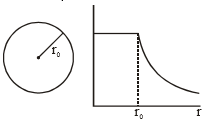# The resistance of the meter bridge AB is given figure is 4. With a cell of emf ε=0.5 V and rheostat resistance Rh= 2the null point is obtained at some point J. When the cell is replaced by another one of emf ε=the same null point J is found for Rh=6. The emfis ;(a) 0.6 V (b) 0.5 V (c) 0.3 V (d) 0.4 V

## Question ID - 50312 :- The resistance of the meter bridge AB is given figure is 4. With a cell of emf ε=0.5 V and rheostat resistance Rh= 2the null point is obtained at some point J. When the cell is replaced by another one of emf ε=the same null point J is found for Rh=6. The emfis ;(a) 0.6 V (b) 0.5 V (c) 0.3 V (d) 0.4 V

3537

Potential gradient with Rh =2is×=L=100cm

Let null point be atcm

Thus=0.5V=……(1)

Now with Rh = 6and at null point…...(2)

Dividing equation (1) by (2) we getthusNext Question :
 The given graph shows variation (with distance r from centre) of :(a) Potential of a uniformly charged sphere (b) Potential of a uniformly charged spherical shell (c) Electric field of uniformly charged spherical shell (d) Electric field of uniformly charged sphere